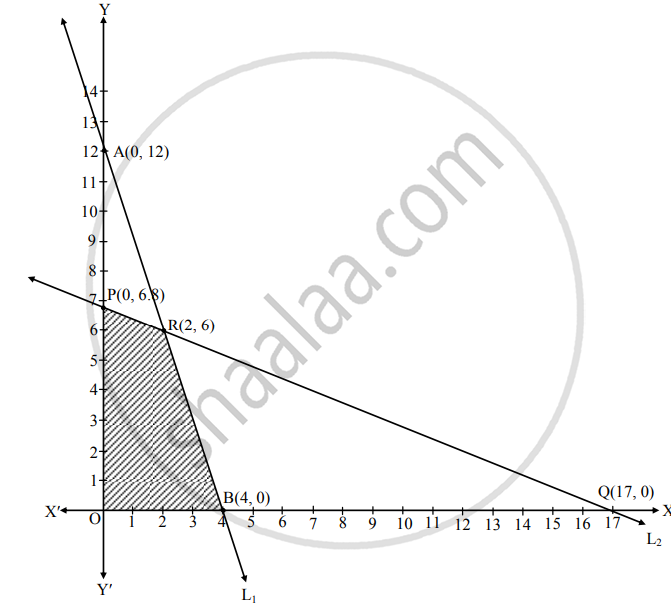# Choose the correct alternative : The maximum value of z = 10x + 6y, subjected to the constraints 3x + y ≤ 12, 2x + 5y ≤ 34, x ≥ 0, y ≥ 0 is. - Mathematics and Statistics

MCQ

Choose the correct alternative :

The maximum value of z = 10x + 6y, subjected to the constraints 3x + y ≤ 12, 2x + 5y ≤ 34, x ≥ 0, y ≥ 0 is.

• 56

• `65

• 55

• 66

#### Solution

Z = 10x + 6y
The given inequalities are 3x + y ≤ 12 and 2x + 5y ≤ 34.
Consider line L1 and L2 where
L1 : 3x + y = 12, L2 : 2x + 5y = 34
For line L1, plot A (0, 12) and B (4, 0)
For line L2, plot P (0, 6.8) and Q (17, 0)
Solving both lines, we get x = 2, y = 6.
The coordinates of origin O (0, 0) satisfies both the inequalities.
∴ The required region is on the origin side of both the lines L1 and L2.
As x ≥ 0, y ≥ 0, the feasible region is in the 1st quadrant.
OBRPO is the required feasible region.
At O (0, 0), Z = 0
At B (4, 0), Z = 10 (4) + 0 = 40
At R (2, 6), Z = 10 (2) + 6 (6) = 56
At P (0, 6.8), Z = 0 + 6 (6.8) = 40.8
The maximum value of Z is 56 and it occurs at R (2, 6).Is there an error in this question or solution?
Chapter 6: Linear Programming - Miscellaneous Exercise 6 [Page 102]

#### APPEARS IN

Balbharati Mathematics and Statistics 2 (Commerce) 12th Standard HSC Maharashtra State Board
Chapter 6 Linear Programming
Miscellaneous Exercise 6 | Q 1.05 | Page 102
Share